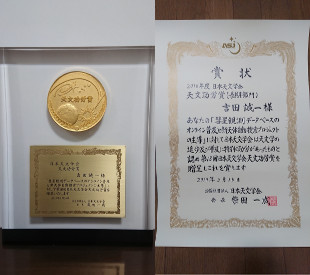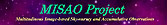#English version Home page Since December 4, 1995 Updated on March 26, 2023## \$B@l6H%H%l!<%@!\$B;#1F!'0f \$B>.OG@1(B32200\$BHV!V5HED@?0l!W(B 2000\$BG/(B7\$B7n(B28\$BF|\$K0f.OG@1(B (32200) 2000 OT2 \$B\$r!"!V5HED@?0l!W\$HL?L>\$7\$FD:\$-\$^\$7\$?!#(B 16.5\$B!A(B20\$BEy\$NL@\$k\$5\$G8+\$(\$F\$\$\$^\$9!#(B\$B5HED@?0l!"EOIt=a0l(B KK\$B%Y%9%H%;%i!<%:(B 2013\$BG/(B6\$B7n(B21\$BF|4)9T(B\$B%U%#%s%i%s%I\$NE7J8;(;o!V(BTahdet ja avaruus\$B!W!J@1\$H1'Ch!K\$N(B2017\$BG/(B2\$B7n9f\$K\$F!";d\$N>R2p5-;v\$r=q\$\$\$FD:\$-\$^\$7\$?!#(B \$B\$B!VWB@14QB,%G!<%?%Y!<%9\$N%*%s%i%\$%sIa5Z\$H?7E7BN<+F0A\:w%W%m%8%'%/%H\$N\$BF|K\E7J83X2q(B 2018\$BG/EY(B \$BE7J88yO+>^!JD94|E*\$J6H@S!K(B\$B\$r^\$\$\$?\$7\$^\$7\$?!#(B## \$BE7J8>pJs(B

• \$BWB@1>pJs(B

• \$BWB@1%+%?%m%0(B (Mar. 26, 2023) [\$B?7Ce>pJs(B]

• \$BN.@172%+%?%m%0(B (Jan. 11, 1998)
• ## \$B4QB,Js9p(B

• \$B5HED@?0l\$NWB@14QB,F|5-(B (Feb. 25, 2022)

• \$BWB@1\$N8wEY4QB,(B (Feb. 25, 2022)
• \$B?7@1!&D6?7@1\$N8wEY4QB,(B (May 7, 2018)
• \$BJQ8w@1\$N8wEY4QB,(B (Nov. 4, 2019)
• \$BN.@14QB,(B (Nov. 21, 2001)

• \$BWB@1\$N(BCCD\$B8wEY4QB,(B (Mar. 5, 2000)
• \$B?7@1!&D6?7@1\$N(BCCD\$B8wEY4QB,(B (Apr. 5, 2000)

• \$B4QB,\$7\$?L@\$k\$\$WB@1%i%s%-%s%0(B
 \$BBh#10L(B -5.2\$BEy(B 2007\$BG/(B1\$B7n(B \$B%^%/%N!<%HWB@1(B(C/2006 P1) \$BBh#20L(B -0.4\$BEy(B 1997\$BG/(B3\$B7n(B \$B%X!<%k(B-\$B%\%C%WWB@1(B(C/1995 O1) \$BBh#30L(B 0.0\$BEy(B 1996\$BG/(B3\$B7n(B \$BI4IpWB@1(B(C/1996 B2) \$BBh#40L(B 2.1\$BEy(B 2007\$BG/(B11\$B7n(B \$B%[!<%`%:WB@1(B(17P) \$BBh#50L(B 3.2\$BEy(B 2005\$BG/(B1\$B7n(B \$B%^%C%/%[%k%DWB@1(B(C/2004 Q2) \$BBh#60L(B 3.3\$BEy(B 2002\$BG/(B4\$B7n(B \$BCSC+(B-\$BD%WB@1(B(153P/2002 C1) 3.3\$BEy(B 2020\$BG/(B7\$B7n(B \$B%K%*%o%\$%:WB@1(B(C/2020 F3) \$BBh#80L(B 3.4\$BEy(B 2004\$BG/(B5\$B7n(B \$B%K!<%HWB@1(B(C/2001 Q4) 3.4\$BEy(B 2018\$BG/(B12\$B7n(B \$B%&%#%k%?%M%sWB@1(B(46P) \$BBh(B10\$B0L(B 3.8\$BEy(B 2015\$BG/(B1\$B7n(B \$B%i%t%8%g%\$WB@1(B(C/2014 Q2) \$BBh(B11\$B0L(B 4.4\$BEy(B 2001\$BG/(B7\$B7n(B \$B%j%K%"WB@1(B(C/2001 A2) \$BBh(B12\$B0L(B 4.9\$BEy(B 2013\$BG/(B11\$B7n(B \$B%i%t%8%g%\$WB@1(B(C/2013 R1)

• \$B4QB,\$7\$?L@\$k\$\$<~4|WB@1%i%s%-%s%0(B
 \$BBh#10L(B 2.1\$BEy(B 2007\$BG/(B11\$B7n(B \$B%[!<%`%:WB@1(B(17P) \$BBh#20L(B 3.3\$BEy(B 2002\$BG/(B4\$B7n(B \$BCSC+(B-\$BD%WB@1(B(153P) \$BBh#30L(B 3.4\$BEy(B 2018\$BG/(B12\$B7n(B \$B%&%#%k%?%M%sWB@1(B(46P) \$BBh#40L(B 5.3\$BEy(B 2008\$BG/(B1\$B7n(B \$B%?%C%H%kWB@1(B(8P) \$BBh#50L(B 5.6\$BEy(B 2010\$BG/(B11\$B7n(B \$B%O!<%H%l! \$BBh#60L(B 5.9\$BEy(B 2006\$BG/(B5\$B7n(B \$B%7%e%t%!%9%^%s(B-\$B%t%!%O%^%sBh#3WB@1(B(73P) \$BBh#70L(B 6.3\$BEy(B 2016\$BG/(B4\$B7n(B \$B%j%K%"WB@1(B(252P) \$BBh#80L(B 6.8\$BEy(B 2018\$BG/(B9\$B7n(B \$B%8%c%3%S%K(B-\$B%D%#%J! \$BBh#90L(B 6.9\$BEy(B 2017\$BG/(B2\$B7n(B \$BK\ED(B-\$B%`%k%3%9(B-\$B%Q%\$%I%%%7%c!<%3%t%!! \$BBh(B10\$B0L(B 7.3\$BEy(B 2017\$BG/(B3\$B7n(B \$B%?%C%H%k(B-\$B%8%c%3%S%K(B-\$B%/%l%5!<%/WB@1(B(41P) \$BBh(B11\$B0L(B 7.5\$BEy(B 2013\$BG/(B11\$B7n(B \$B%(%s%1WB@1(B(2P) \$BBh(B12\$B0L(B 7.7\$BEy(B 1998\$BG/(B1\$B7n(B \$B%F%s%Z%k(B-\$B%?%C%H%kWB@1(B(55P)
• ## \$B8&5f%W%m%8%'%/%H(B

• \$BE7BN2hA|\$+\$i\$N?7E7BNA\:w(B
MISAO\$B%W%m%8%'%/%H(B• ## \$B<9I.!&O":\(B

• \$BBgWB@1!"8=\$k!#(B\$B5HED@?0l!"EOIt=a0l(B KK\$B%Y%9%H%;%i!<%:(B 2013\$BG/(B6\$B7n(B21\$BF|4)9T(B
• \$B7n4)@1%J%S(B \$B5HED@?0l\$N;kE7(B2022\$BG/(B3\$B7n9f(B 2021\$BG/\$NG/\$N@%\$O!"%l%J!<%IWB@1(B(C/2021 A1)\$B\$NOCBj\$G;}\$A\$-\$j\$H\$J\$C\$?!#(B \$BM=A[30\$N%"%&%H%P!<%9%H\$r5/\$3\$7!"FMG!\$H\$7\$FL@\$k\$/\$J\$C\$?%l%J!<%IWB@1\$O!"%/%j%9%^%9\$+\$iG/KvG/;O\$K\$+\$1\$F!"B?\$/\$NWB@1%U%!%s\$r3Z\$7\$^\$;\$F\$/\$l\$?!#(B \$B%l%J!<%IWB@1%"%&%H%P!<%9%H!*(B
• ## \$B%\$%Y%s%H(B

• \$BBh(B45\$B2s(B \$BWB@12q5D(B in \$B\$5\$\$\$?\$^Bg5\(B\$B"#(B \$BF|;~!'(B2015\$BG/(B6\$B7n(B6\$B!A(B7\$BF|!JEZF|!K(B \$B"#(B \$B2q>l!'\$5\$\$\$?\$^;T1'Ch7`>l!J#J#RBg5\1X@>8}ELJb#3J,!K(B \$B"#(B \$BFbMF!'(B \$B4pD49V1i!VBgWB@1\$,8=\$l\$k\$^\$G!W(B \$B5~ET;:6HBg3X65\$B>7BT9V1i!V4QB,5!4o3+H/\$HBg5,LO%5!<%Y%\$!!!](B CCD \$B%+%a%i<+ \$BT"N)Cf1{BgU\E7J88&5f=j=uM}65\$BF~Lg9V:B!V4Q\$FB,\$kWB@19V:B!W(B \$BLgED7r0l;a!":4F#1Q5.;a!">.NSN4CK;a!"1J0f2B\$B@16u2;3Z%f%K%C%H!V%"%/%"%^%j%s!W%i%\$%V(B \$B"(%l%;%W%7%g%s!J:)?F2q!K(B
• ## \$BE7J8%=%U%H%&%'%"(B

• \$BE7BN2hA|<+F08!::%7%9%F%`(B
PIXY\$B%7%9%F%`(B2 (Jan. 2, 2005)

• Comet for Windows (June 16, 2007)

• loneos.phot\$B\$N8!:w%D!<%k(B (June 11, 2003)

• \$B%K%e!<%i%k%M%C%H%o!<%/\$K\$h\$kWB@14QB,%9%1%8%e!<%i(B (July 21, 2004)
• \$B%K%e!<%i%k%M%C%H%o!<%/\$K\$h\$k%a%7%(E7BN4QB,%9%1%8%e!<%i(B (Jan. 22, 1998)

• \$BE7BN2hA|MQ\$*M7\$S%U%#%k%?!<(B Filters for Windows (June 6, 1998)
• ## \$BE7J8;qNA(B

• ICQ\$B%U%)!<%^%C%H\$N2r@b(B (Dec. 29, 2007)
• \$B8wEY%7%9%F%`!&?'JQ49\$N8x<0=8(B (Jan. 1, 2010)
• ## \$BE7J88&5f(B

###\$BWB@18&5f(B• \$B<~4|WB@1\$NH/8+\$H!"\$=\$NL?L>\$K\$D\$\$\$F\$N9M;!(B (Oct. 9, 2022)
• C/2001 OG108\$B\$N3K8wEY\$N@bL@\$G\$-\$J\$\$8:8w(B (Dec. 12, 2005)
• 3\$B\$D\$N?7\$7\$\$\$*\$&\$7J#9g72\$N8uJdE7BN(B (Nov. 28, 2005)
• NASA\$B%G%#!<%W%\$%s%Q%/%H!'%"%^%A%e%"4QB,\$B%F%s%Z%kBh#1WB@1\$NEy5i\$O!)(B (Aug. 9, 2005)
• \$BCO>e\$+\$i\$N%(%s%1WB@1\$N3KI=LL\$N4QB,(B (July 21, 2005)
• \$B4qL/\$JWB@1(B C/2003 T4 ( LINEAR ) (June 21, 2005)
• \$B8O3i\$7\$?WB@1%U%!%(%H%s\$N%@%9%H%H%l%\$%k(B (June 12, 2005)
• 162P/2004 TU12\$B\$N0lD>@~\$NHx(B (Nov. 18, 2004)
• ###\$B%+%?%m%08&5f(B• \$B6d2O\$NEy5iJL!&%+%?%m%0JL\$NE}7W(B (May 12, 2003)
• 2MASS\$B\$G8!=P\$5\$l\$?WB@1\$N8!>Z(B (May 3, 2003)
• ###\$B%3%i%`(B• Landolt\$BI8=`@1\$NJQ\$o\$C\$?@1(B (May 11, 2003)
• C/1996 Q1 ( Tabur ) \$B\$NH/2;(B (Dec. 12, 1996)
• ## \$B9V1i!&H/I=(B

###\$BWB@1(B• \$BBh(B49\$B2sWB@12q5D(B (2019\$BG/(B6\$B7n(B15\$B!A(B16\$BF|(B)
2018\$BG/\$N (June 16, 2019)
• \$BBh(B48\$B2sWB@12q5D(B (2018\$BG/(B6\$B7n(B16\$B!A(B17\$BF|(B)
2017\$BG/\$N (June 18, 2018)
• \$BBh(B47\$B2sWB@12q5D(B (2017\$BG/(B6\$B7n(B10\$B!A(B11\$BF|(B)
2016\$BG/\$N (June 14, 2017)
• \$BBh(B46\$B2sWB@12q5D(B (2016\$BG/(B6\$B7n(B18\$B!A(B19\$BF|(B)
2015\$BG/\$N (June 23, 2016)
• \$BBh(B45\$B2sWB@12q5D(B (2015\$BG/(B6\$B7n(B6\$B!A(B7\$BF|(B)
2014\$BG/\$N (June 11, 2015)
• \$BBh(B44\$B2sWB@12q5D(B (2014\$BG/(B6\$B7n(B7\$B!A(B8\$BF|(B)
2013\$BG/\$N (June 15, 2014)
• \$BBh(B43\$B2sWB@12q5D(B (2013\$BG/(B6\$B7n(B15\$B!A(B16\$BF|(B)
2012\$BG/\$N (June 29, 2013)
• \$BBh(B42\$B2sWB@12q5D(B (2012\$BG/(B6\$B7n(B2\$B!A(B3\$BF|(B)
2011\$BG/\$N (June 8, 2012)
• \$BBh(B41\$B2sWB@12q5D(B (2011\$BG/(B7\$B7n(B16\$B!A(B17\$BF|(B)
2010\$BG/\$N (July 27, 2011)
• \$BBh(B40\$B2sWB@12q5D(B (2010\$BG/(B4\$B7n(B24\$B!A(B25\$BF|(B)
2009\$BG/\$N (July 27, 2011)
• \$BBh(B39\$B2sWB@12q5D(B (2009\$BG/(B4\$B7n(B18\$B!A(B19\$BF|(B)
2008\$BG/\$N (July 27, 2011)
• \$BBh(B38\$B2sWB@12q5D(B (2008\$BG/(B5\$B7n(B17\$B!A(B18\$BF|(B)
2007\$BG/\$N (July 27, 2011)
• \$BBh(B37\$B2sWB@12q5D(B (2007\$BG/(B4\$B7n(B7\$B!A(B8\$BF|(B)
2006\$BG/\$N (July 27, 2011)
• \$BBh(B36\$B2sWB@12q5D(B (2006\$BG/(B3\$B7n(B18\$B!A(B19\$BF|(B)
2005\$BG/\$N (July 12, 2006)
• \$BBh(B35\$B2sWB@12q5D(B (2005\$BG/(B3\$B7n(B19\$B!A(B20\$BF|(B)
2004\$BG/\$N (July 19, 2005)
• \$BBh(B34\$B2sWB@12q5D(B (2004\$BG/(B3\$B7n(B6\$B!A(B7\$BF|(B)
2003\$BG/\$N (Aug. 31, 2004)
• \$BBh(B34\$B2sWB@12q5D!!(B2\$BBgWB@14QB,9V:B(B (2004\$BG/(B3\$B7n(B6\$B!A(B7\$BF|(B)
2\$BBgWB@1\$N35MW\$H8=67(B (Aug. 31, 2004)
• \$B\$D\$/\$P@1\$N2q2qJs!V@16u\$D\$/\$P!W(BNo.152 (1998\$BG/(B1\$B7n9f(B)
\$B%F%s%Z%k(B-\$B%?%C%H%kWB@1\$r8+\$h\$&!*(B (Jan. 31, 1998)
• \$BBh(B61\$B2sEl5~6a9YCO6hN.@14QB, \$B%X!<%k(B-\$B%\%C%WWB@1\$K\$D\$\$\$F(B (Mar. 26, 1997)
• \$BBh(B58\$B2sEl5~6a9YCO6hN.@14QB, \$BI4IpWB@1\$r8+\$h\$&(B (Nov. 12, 1996)
• ###\$BN.@1(B• \$BBh(B62\$B2sEl5~6a9YCO6hN.@14QB, \$BN.@172%+%?%m%0\$K\$D\$\$\$F(B (June 15, 1997)
• ###\$B%=%U%H%&%'%"(B• 1996\$BG/WB@12F\$N3X9;(B (1996\$BG/(B8\$B7n(B31\$BF|!A(B9\$B7n(B1\$BF|(B)
\$BE7J8E}9g4D6-(B Comet for Windows [\$BH/I=;~G[IU;qNA(B] (Nov. 11, 1996)
\$BE7J8E}9g4D6-(B Comet for Windows [\$B=8O?7G:\869F(B] (Mar. 8, 1997)
• \$BBh(B26\$B2sWB@12q5D(B (1996\$BG/(B3\$B7n(B30\$BF|!A(B31\$BF|(B)
Comet for Windows: \$B8wEY%G!<%?4IM}!&8wEY<07W;;%=%U%H(B (Nov. 11, 1996)
• ###\$B\$=\$NB>(B• \$B0q>kE7J80&9% \$B4QB,7k2L\$r@\$3&\$X!*(B \$B%\$%s%?!<%M%C%H\$HH~%9%?!<(B (Nov. 11, 1996)
•